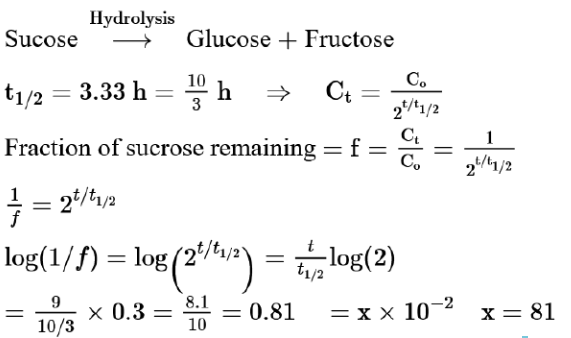# Sucrose hydrolyses in acid solution into glucose and fructose followingQuestion:

Sucrose hydrolyses in acid solution into glucose and fructose following first order rate law with a half-life of $3.33 \mathrm{~h}$ at $25^{\circ} \mathrm{C}$. After $9 \mathrm{~h}$, the fraction of sucrose remaining is $\mathrm{f}$. The value of $\log _{10}\left(\frac{1}{\mathrm{f}}\right)$ is____________ $\mathrm{mat} \times 10^{-2}$ (Rounded off to the nearest integer) [Assume: $\ln 10=2.303, \ln 2=0.693$ ]

Solution:

(81)